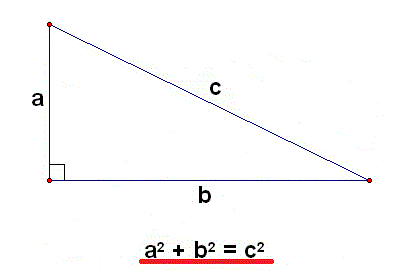Detailed Right Triangle (◺) Pythagorean Theorem CalculationA Step By Step Pythagorean Theorem Calculator

An online detailed geometry pythagoreas calculator along with step by step calculation.

Step by Step calculationPythagorean Theorem Formula

a2 + b2 = c2

For example: To Find A, Enter b=7, c=9, Answer is A= 5.66)The Pythagorean Theorem is the statement that the sum of (the areas of) the two small squares equals (the area of) the big one.

In math algebraic terms, a2 + b2 = c2 where c is the hypotenuse while a and b are the legs of the triangle.Related Calculator

Distance Between Two Points

Distance Between Two MidPoints

Geometric Progression Calculator

Arithmeic Progression CalculatorMore Calculators

Short Distance Between Two Lines

Geometric Mean Calculator

More >>Age Calculators ►

Horse Age Calculator , Cow Age Calculator , Cat Age Calculator , Dog Age Calculator, Human Age Calculator 100 Days Old Calculator Date Difference Calculator